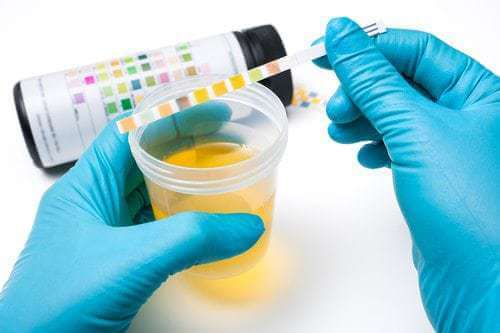# What Are the Odds of Failing a Drug Test?Topics

Last updated:
07/10/2021

#### What are the odds that a person who tests positive for an illicit drug is actually using that drug?

That is a vital question in pain management and the opioid crisis, because millions of pain patients undergo drug tests regularly. The answer is not just a matter of looking at the accuracy of the test.

In a simple situation, like a toss of a coin or a roll of a die, computing the probability of an outcome is elementary. Most people realize that a toss of a coin has an equal chance of coming up heads or tails.

But drug testing is not as simple. It is an example of a conditional probability. A drug test that is 95% accurate will not find drug users 95% of the time. That is because the test is applied to both drug users and non-users. We have use a calculation known as Bayes’ Theorem to determine the real probabilities.

#### How is Bayes Theorem applied in drug testing?

Bayes’ Theorem calculates the probability of one event happening given that another event has already happened. In terms of drug testing, this means the probability that a randomly selected person who has a positive test is in fact a drug user.

To perform the calculations, we need to know two things:

1) the accuracy of the drug test

2) the probability that a randomly selected person is a drug user, or the “base rate” at which drug use occurs in the population at large.

#### What percent of drug tests are inaccurate?

The accuracy of drug tests varies widely. A 2010 study estimated that drug tests generally produce false-positive results in 5% to 10% of cases and false negatives in 10% to 15% of cases.

Data on the base rate of drug use also varies. The CDC claims as many as 25% of chronic pain patients develop signs of opioid use disorder. However, a Cochrane review found addiction in less than 2%…